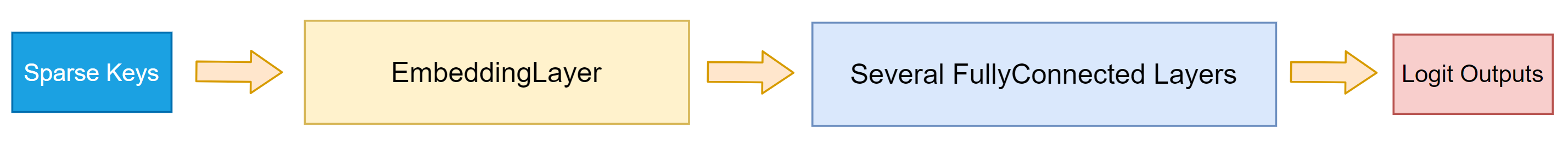# Demo model using Dense Embedding Layer

This file demonstrates how to build a DNN model with dense embedding layer, where no reduction will be conducted intra each slot (feature-filed), with TensorFlow and SparseOperationKit.

You can find the source codes in sparse_operation_kit/documents/tutorials/DenseDemo/.

## requirements

python modules: cupy, mpi4py, nvtx

## model structure

This demo model is constructed with a dense embedding layer and 7 fully connected layers, where the former 6 fully connected layers have 1024 output units, and the last one has 1 output unit.## steps

### Generate datasets

This commands will generate a dataset randomly. By default, its filename is data.file, you can specify the output filename by adding --filename=XXX when running this command.

$python3 gen_data.py \ --global_batch_size=65536 \ --slot_num=100 \ --nnz_per_slot=10 \ --iter_num=30  ### Split the whole dataset into multiple shards When MPI is used, we’d like to let each CPU process have its own datareader, and each datareader reads from different data source. Therefore the whole dataset is splited. The splited files will be saved with name: save_prefix[split_id].file, for example, data_0.file, data_1.file. And the samples in each shard are linearly arranged. For instance, the whole samples is [s0, s1, s2, s3, s4, s5, s6, s7], when they are splited into 4 shards, each shard owns 2 samples, which is [s0, s1], [s2, s3], [s4, s5], [s6, s7], respectively. $ python3 split_data.py \
--filename="./data.file" \
--split_num=8 \
--save_prefix="./data_"


### Run this demo writen with TensorFlow

This is a model parallelism demo implemented by tf methods.

$mpiexec -n 8 --allow-run-as-root \ python3 run_tf.py \ --data_filename="./data_" \ --global_batch_size=65536 \ --vocabulary_size=8192 \ --slot_num=100 \ --nnz_per_slot=10 \ --num_dense_layers=6 \ --embedding_vec_size=4 \ --optimizer="adam" \ --data_splited=1  ### Run this demo writen with SOK + MirroredStrategy $ python3 run_sok_MirroredStrategy.py \
--data_filename="./data.file" \
--global_batch_size=65536 \
--max_vocabulary_size_per_gpu=8192 \
--slot_num=100 \
--nnz_per_slot=10 \
--num_dense_layers=6 \
--embedding_vec_size=4 \


### Run this demo writen with SOK + MultiWorkerMirroredStrategy + MPI

Add --oversubscribe to mpiexec if there is not enough slots.

$mpiexec -n 8 --allow-run-as-root \ python3 run_sok_MultiWorker_mpi.py \ --data_filename="./data_" \ --global_batch_size=65536 \ --max_vocabulary_size_per_gpu=8192 \ --slot_num=100 \ --nnz_per_slot=10 \ --num_dense_layers=6 \ --embedding_vec_size=4 \ --data_splited=1 \ --optimizer="adam"  ### Run this demo writen with SOK + Horovod $ horovodrun -np 8 -H localhost:8 \
python3 run_sok_horovod.py \
--data_filename_prefix="./data_" \
--global_batch_size=65536 \
--max_vocabulary_size_per_gpu=1024 \
--slot_num=100 \
--nnz_per_slot=10 \
--num_dense_layers=6 \
--embedding_vec_size=4 \